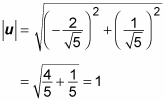##### Calculus II For DummiesEvery nonzero vector has a corresponding unit vector, which has the same direction as that vector but a magnitude of 1. To find the unit vector u of the vector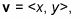you divide that vector by its magnitude as follows: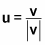Note that this formula uses scalar multiplication, because the numerator is a vector and the denominator is a scalar.

A scalar is just a fancy word for a real number. The name arises because a scalar scales a vector — that is, it changes the scale of a vector. For example, the real number 2 scales the vector v by a factor of 2 so that 2v is twice as long as v.

As you may guess from its name, the unit vector is a vector.

For example, to find the unit vector u of the vector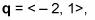you first calculate its magnitude |q|: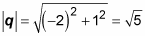Now use the previous formula to calculate the unit vector: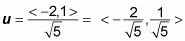You can check that the magnitude of resulting vector u really is 1 as follows: